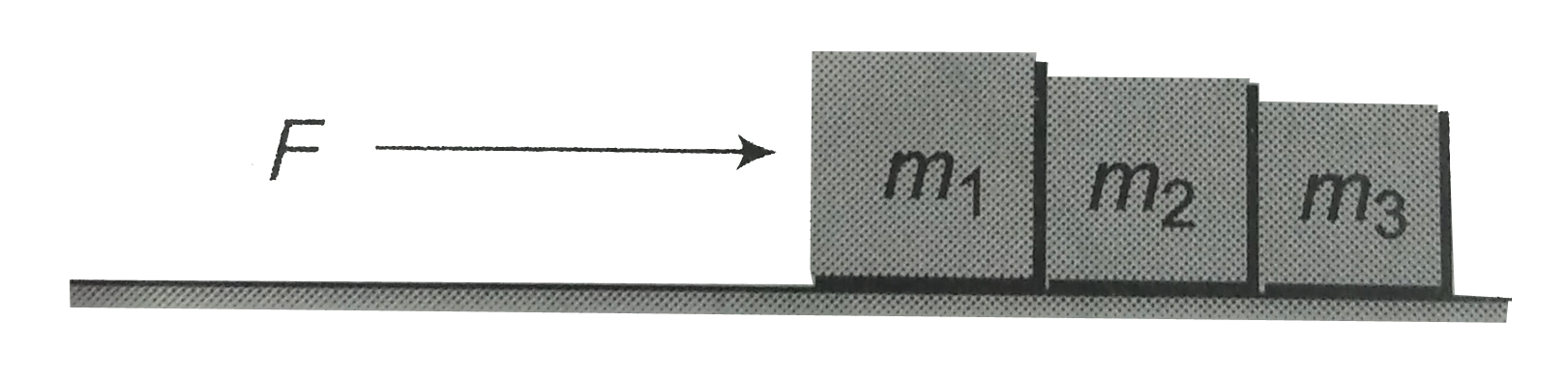# Three block of masses m_(1),m_(2) and m_(3) kg are placed in contact with each other on a frictionless table. A force F is applied on the heaviest

10 views
in Physics
Three block of masses m_(1),m_(2) and m_(3) kg are placed in contact with each other on a frictionless table. A force F is applied on the heaviest mass m_(1), the acceleration of m_(2) will beA. (F)/(m_(1))
B. (F)/((m_(1)+m_(2))
C. (F)/((m_(1)-m_(2))
D. (F)/((m_(1)+m_(2)+m_(3))

by (37.3k points)
Correct Answer - D